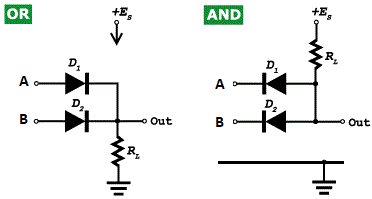# Logic Gates Experiment (AND and OR gates)

Aim : To verify the truth tables of OR and AND gates using diodes.

Apparatus : Two diodes (ex: IN 4001), 5k Ω resistor, a 5V dc source, voltmeter (0-5V); SPDT toggle switch; tap keys, etc.

Theory : A switch that can be opened or closed is known as a gate and the two positions of the switch can be described as gate open and gate closed. Thus a gate circuit has two possible states described as ON or OFF, True or False, Yes or No, High or Low and so on. These two states can be represented by the binary digits 1 and 0.
The circuits which are used to perform switching action are known as logic circuits or logic gates. A logic gate may have a number of possible inputs and the output signal will be obtained only if the input signal meet certain specific conditions.

A gate circuit which provides an output when both the inputs are ON is known as AND gate. A gate circuit which provides an output when either one of the input is ON is called OR gate.

Levels of ± 5V and 0V are often used to represent high (1) and low (0) in logic circuits. The truth table for AND and OR gates are given. (A and B represent inputs and Y represents the output)Symbol and Truth TableCircuit Diagram, OR and AND
Procedure :

(i) To verify the truth table of AND gate

Connections are made as shown in the figure. A and B are the input gates and O is the output terminal. The input terminals A and B are earthed by putting A in contact with T2 and B with T4 of the toggle switch. So, the inputs A = 0 and B = 0. Since both the diodes are in forward bias, they conduct current through the resistor R. The output voltage Y is measured between the terminal 0 and the earthed point by the voltmeter. It is found to be 0V. (Actually 0.7V will be developed across the silicon diode and 0.3V across the germanium diode. Hence the output will be 0.7V or 0.3V. But this can be neglected in comparison with the large input voltage V = 5V). Thus it is shown that when A = 0 and B = 0, then Y = 0.

A positive voltage V = 5V is applied to A by putting A in contact with T1. (The negative terminal of the source is earthed). The terminal B is earthed. So, the inputs A = 1 and B = 0. The diode D1 will be reverse biased, because its cathode is having a voltage equal to +5V. Due to reverse biasing, the diode D1 will stop conducting. But the diode D2 is forward biased and will be conducting through the resistor R. As before, the output Y is measured. The output will be zero as seen earlier. Hence, when A = 1 and B = 0, then Y = 0.

The experiment is repeated applying +5V to the input terminal B and keeping the input terminal A earthed. The output is measured. It is found that the output Y = 0V. Thus, when A = 0, B = 1, then Y = 0.

The experiment is also repeated applying +5V to each of the input terminals A and B by putting A in contact with T1 and B in contact with T3. So, A = 1 and B = 1. Now all the diodes are in reverse bias and hence will stop conducting. The output voltage Y is measured. It is seen that output voltage is 5V. Thus, when A = 1 and B = 1, then Y = 1. Thus the truth table of the AND gate is verified. [The SPDT toggle switch is used to apply the inputs to A and B or to ground A and B].

(ii) To verify the truth table of OR gate

Connections of the OR gate is made as shown in the figure. The keys K1, and K2 are kept open. So, the inputs A = 0 and B = 0. The output voltage across R is measured. Since the diodes do not conduct, it is seen that the output Y = 0. Thus, when A = 0 and B = 0, then Y = 0.

The key K1 is closed and K2 is kept open. So A = 1 and B = 0. Since D1 is in forward bias, it conducts current through R. The output voltage Y across R is measured. It is found that the output voltage is almost 5V. Hence, Y = 1. Thus it is shown that when A = 1 and B= 0, then Y = 1.
The experiments is repeated by closing the key K1 and keeping K2 open. It is seen that Y = 1. Thus, when A = 0 and B = 1, then Y = 1.

The experiment is also repeated with K1 and K2 closed. In this case also Y = 1. This shows that when A = 1 and B = 1, then Y = 1. Thus the truth table of OR gate is verified.

Results : The truth tables of AND and OR gates are verified.